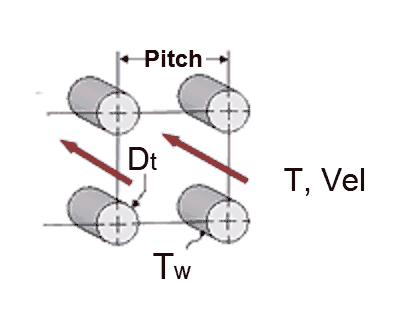Contents - Index

Tube_BundleProcedure Tube_Bundle(Fluid\$, T, P, G\$, Vel, D_t, Pitch, T_w, L_t, D_hp: DELTAP, h_bar, Re, f,  Nusselt) returns the average heat transfer coefficient and the pressure drop for a specified fluid velocity (Vel)  through an ideal tube bundle having either square or triangular tube layout.  Flow can be vertical (up or down) or horizontal.as the literature shows little difference between these flow arrangements.  Properties are evaluated at the bulk temperature T and pressure P, for all built-in fluids except brines, for which the concentration in mixtures with water is provided in % in place of pressure.  Units of the inputs and outputs depend upon the unit settings in EES.

Inputs:

Fluid\$ can be any fluid in the EES database.  The fluid can be an ideal gas, a real fluid, a brine, or a fluid from the Solid-Liquid property library.

T - the bulk temperature of the fluid  in  [C], [K], [F], or [R].

P -  pressure can be in [Pa], [kPa], [bar], [MPa], [atm], or [psia].  (Note that for brines, this parameter provides the concentration in %)

G\$ - a string or string variable that is set to either 'Square' or 'Triangular', referring to the arrangement of the tubes in the tube bundle

Vel  -  average velocity of the fluid in the tube bundle.

D_t -  diameter of the tubes in [m] or [ft]

T_w - temperature of the wall of the tubes in  [C], [K], [F], or [R].  (This input is used to determine a wall temperature correction factor. )

Pitch  -distance between tube centers in a square or triangular arrangement [m] or [ft]

L_t - length of the tubes  in [m] or [ft]

D_hp - mean hydraulic diameter of the tube bundle defined as 4*(total flow area)/(p*N*D_t+p*D_s)

where N is the number of tubes and D_s is the inside diameter of the shell enclosing the tubes

Outputs: (note that it is not necessary to provide all of the outputs.  See below.)

DELTAP - pressure difference between the inlet and outlet of the tube bundle (assuming no baffles) calculated as DELTAP=f*rho*Vel^2/2*L_t/D_hp

h_bar -  average heat transfer coefficient in [W/m^2-K] or [Btu/hr-ft^2-R]

Re - Reynolds number [-]

f -  friction factor [-]

Nusselt_T - Nusselt number (determined for a constant temperature wall) [-]

Notes

Longitudinal flow can be classified as either internal or external flow.

This procedure  calculates the Reynolds and Prandtl numbers, the wall temperature correction factor (wtc) and the ratio Pitch/D_t then calls  Tube_Bundle_ND

The procedure will determine if the flow is laminar or turbulent and apply the appropriate correlation.  Fully-developed flow is assumed.

Inputs and outputs in the Call statement are separated with a list separator character, which is a comma in the US system and a semicolon in the European system.

Example:

\$UnitSYstem SI C kPa kJ mass deg

D_t=0.05 [m]

Pitch=0.075 [m]

F\$='Water'

T=60 [C]

P=1000 [kPa]

G\$='SQUARE'

T_w=90 [C]

L_t=2 [m]

D_hp=0.1 [m]

Vel=0.05 [m/s]

Call tube_bundle(F\$, T, P, G\$, Vel, D_t, Pitch, T_w, L_t, D_hp:DELTAP, h_bar, Re, f,  Nusselt)

{Solution:

DELTAP=0.0008652 [kPa]

f=0.03519

h_bar=530.4 [W/m^2-K]

Nusselt=75.92

Re=9827

}

NOTE:  Starting with version 9.513, it is not necessary to supply all of the outputs to a Procedure.  If, for example, you only want to return output h_bar, the call statement can appear as:

Call tube_bundle(F\$, T, P, G\$, Vel, D_t, Pitch, T_w, L_t, D_hp: , h_bar, , , )